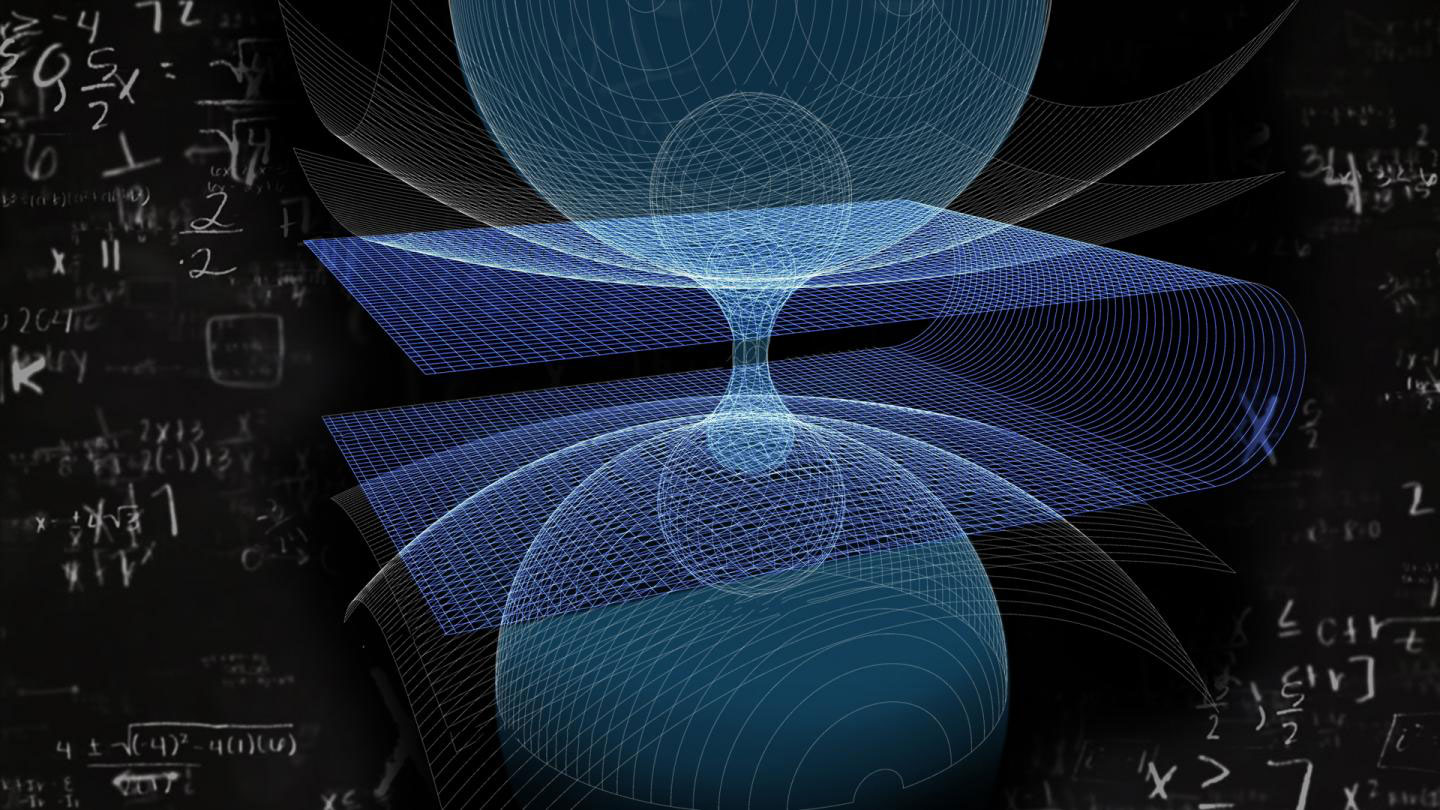# The Shape of a Wormhole Calculated by a ScientistSHARE

A recent research, which was published in the journal Physics Letters B, shows how the shape of any symmetrical wormhole can be described using its wave spectrum. A wormhole is a type of black hole that in theory can act as a portal connecting any two points in time and space, and thanks to this study, the scientists could expand their knowledge about the physics and characteristics of these mysterious objects.

How to determine the size and shape of a wormhole

According to our current understanding of the universe, the existence of wormholes, strange curvatures in time and space, is theoretically possible. At the present moment, the astrophysicists do not know how to determine the size and shape of such objects. However, the recent study, which was conducted by Roman Konoplya, a research assistant at the RUDN Institute of Gravitation and Cosmology, explains the way we can calculate the shape of a wormhole using observable physical characteristics.

The only property of a wormhole that we can observe indirectly is a red shift, which is a downward shift in the frequency of gravitational waves in their way from an object. In his study, Konoplya used the red shift value and the range of gravitational waves in high frequencies to calculate both the shape and mass of a wormhole, according to geometrical and quantum mechanical assumptions.

How was the study conducted

Konoplya based his research on the mathematical model of a spherically symmetrical Morris-Thorne wormhole, which is a black hole that connects two points in time and space and, in theory, allows the movement between these two points. Then, an existing mathematical model was used to depict the bottleneck of the wormhole (the narrowest point between the entrance and exit of the wormhole). The scientist first mathematically described the way the wave range of any symmetrical wormhole can be used to determine its shape, solving what we know in general terms as the opposite problem. Then he managed to establish an equation that allows to calculate the geometrical shape for a wormhole, using quantum mechanical approximation.

SHARE